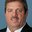Question-and-Answer Resource for the Building Energy Modeling Community
Get started with the Help page

Unit inducing mistake when you set a Cooling Tower in OpenStudio / EnergyPlusOnly to inform other users, if you are setting Cooling Tower, if you use Evaporation Loss Mode: Loss Factor, you will need to set Evaporation Loss Factor.

The unit showed is percent/K. So, InputOuputReference indicates a typical value from 0,15 to 0,27 [percent/K].

But you need to set in program 0,0015 to 0,0027 to result in reasonable results. In other words, you need "to do the percent" before you set this parameter. If not, the results are unreals.

edit retag close merge delete

Sort by » oldest newest most votedYour right. The documentation does show units of percent. Same for the next field of drift loss percent. A review of the code shows that the Evaporation Loss Factor field is currently a fraction, not percent, of water flow rate.

The IDD shows the same information described in the Input Output Reference document:

N19, \field Evaporation Loss Factor
\type real
\units percent/K
\note Rate of water evaporation from the cooling tower and lost to the outdoor air [%/K]
\note Evaporation loss is calculated as percentage of the circulating condenser water rate
\note Value entered here is percent-per-degree K of temperature drop in the condenser water
\note Typical values are from 0.15 to 0.27 [%/K].
\default 0.2
N20, \field Drift Loss Percent
\type real
\default 0.008
\units percent
\note Rate of drift loss as a percentage of circulating condenser water flow rate
\note Typical values are between 0.002 and 0.2% The default value is 0.008%

And the code where these data are read in shows:

SimpleTower( TowerNum ).UserEvapLossFactor = NumArray( 19 ); //  N11 , \field Evaporation Loss Factor
SimpleTower( TowerNum ).DriftLossFraction = NumArray( 20 ) / 100.0; //  N12, \field Drift Loss Percent
more